NEET  >  Test: Thermochemistry

# Test: Thermochemistry

Test Description

## 30 Questions MCQ Test Chemistry Class 11 | Test: Thermochemistry

Test: Thermochemistry for NEET 2023 is part of Chemistry Class 11 preparation. The Test: Thermochemistry questions and answers have been prepared according to the NEET exam syllabus.The Test: Thermochemistry MCQs are made for NEET 2023 Exam. Find important definitions, questions, notes, meanings, examples, exercises, MCQs and online tests for Test: Thermochemistry below.
Solutions of Test: Thermochemistry questions in English are available as part of our Chemistry Class 11 for NEET & Test: Thermochemistry solutions in Hindi for Chemistry Class 11 course. Download more important topics, notes, lectures and mock test series for NEET Exam by signing up for free. Attempt Test: Thermochemistry | 30 questions in 45 minutes | Mock test for NEET preparation | Free important questions MCQ to study Chemistry Class 11 for NEET Exam | Download free PDF with solutions
 1 Crore+ students have signed up on EduRev. Have you?
Test: Thermochemistry - Question 1

### For which of the following change ΔH ≠ ΔE ?

Detailed Solution for Test: Thermochemistry - Question 1

∆H = ∆U+∆ngRT
For ∆H not equal ∆U, ∆ng should not equal zero.
This happens only in option d, where ∆ng  = -2

Test: Thermochemistry - Question 2

### ΔrH of which of the following reactions is zero ?

Detailed Solution for Test: Thermochemistry - Question 2

In equation d, H+(aq) is formed from H2(g). Enthalpy of formation of both entity is considered to be zero. So, ∆rH (∆Hproduct - ∆Hreactant) is zero.

Test: Thermochemistry - Question 3

### How much heat will be required at constant pressure to form 1.28 kg of CaC2 from CaO(s) & C(s) ? Given : ΔfH°(CaO, s) = -152 kcal/mol ΔfH°(CaC2, s) = -14 kcal/mol ΔfH°(CO, g) = -26 kcal/mol

Detailed Solution for Test: Thermochemistry - Question 3

Reaction is CaO + C → CaC2+CO
1.28 kg of CaC2 means 20 mole CaC2 is needed
For 1 mole dH=-26-14-(-152)=112
For 20 Mole 112×20=2240

Test: Thermochemistry - Question 4

50.0 mL of 0.10 M HCl is mixed with 50.0 mL of 0.10 M NaOH. The solution temperature rises by 3.0°C Calculate the enthalpy of neutralization per mole of HCl. [take proper assumptions]

Detailed Solution for Test: Thermochemistry - Question 4

No. of moles of HCl = 5 millimoles
No. of moles of NaOH = 5 millimoles
Mass of solution mixed = 50 gm+50 gm=100 gm
ΔH=−cmΔT(c=4.18 kJKg−1)
⇒ ΔH=−4.18×0.1×3
⇒ ΔH=−1.254 kJ (For 5 millimoles of water formed)
For 1 mole water = −1.254/5×10−3
=−2.5×102kJ

Test: Thermochemistry - Question 5

The enthalpy of neutralisation of a weak acid in 1 M solution with a strong base is -56.1 kJ mol-1. If the enthalpy of ionization of the acid is 1.5 kJ mol-1 and enthalpy of neutralization of the strong acid with a strong base is -57.3 kJ equiv-1, what is % ionization of the weak acid in molar solution (assume the acid to be monobasic) ?

Detailed Solution for Test: Thermochemistry - Question 5

The correct answer is option C
Ideally, the enthalpy of neutralization should be - 57.3 K.J + 1.5 K.J = - 55.8 KJ.
But it is - 56.1 KJ.
∴ Energy used for neutralization
= 57.3 - 56.1 = 1.2 KJ
∴ Percent ionization of weak acid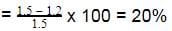∴ % of weak acid in solution = 20%

Test: Thermochemistry - Question 6

For the allotropic change represented by the equation C (graphite) → C (diamond), ΔH = 1.9 kJ. If 6 g of diamond and 6 g of graphite are separately burnt to yield CO2, the heat liberated in first case is

Detailed Solution for Test: Thermochemistry - Question 6

∆H given in the question is for one mole of C (g). If 6 gm of diamond and graphite are burnt in oxygen then the C (diamond) will first convert to graphite and then it will form CO2. While C (graphite) will directly form CO2. So due to the conversion of diamond into graphite, we will get extra heat. And since we have 6gm (0.5 mol) of diamond, so the heat released will be 0.5×1.9 kJ or 0.95 kJ more than the second case.

Test: Thermochemistry - Question 7

If x1, x2 and x3 are enthalpies of H - H, O = O and O - H bonds respectively, and x4 is the enthalpy of vaporisation of water, estimate the standard enthalpy of combustion of hydrogen

Detailed Solution for Test: Thermochemistry - Question 7

Combustion of hydrogen:
H2 + ½ O2→H2O (H−O−H)
As water contains two O−H bonds.
So, combustion enthalpy of hydrogen is:
ΔH= Bond energy of reactant − Bond energy of the product − Enthalpy of vaporization.
ΔH=x1+22−2x3−x4

Test: Thermochemistry - Question 8

NH3(g) + 3Cl2(g)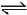NCl3(g) + 3HCl(g) ; -ΔH1

N2(g) + 3H2(g)2NH3(g) ; ΔH2

H2(g) + Cl2(g)2HCl(g) ; ΔH3

The heat of formation of NCl3 (g) in the terms of ΔH1, ΔH2 and ΔH3 is

Detailed Solution for Test: Thermochemistry - Question 8

The formation of NCl3 be like
½ N2 + 3/2Cl2 ⇋ NCl3
We can see that for this setup, we need to have eqn (ii) divided by 2, reversing eqn (iii) and multiplying it by 3/2 and then adding all these to equation (i).
So option a is correct.

Test: Thermochemistry - Question 9

The enthalpy of neutralisation of HCl and NaOH is -57 kJ mol-1. The heat evolved at constant pressure (in kJ) when 0.5 mole of H2SO4 react with 0.75 mole of NaOH is equal to

Detailed Solution for Test: Thermochemistry - Question 9

-57 kJ of heat evolved when 1 mole of NaOH reacted with an acid.
We have 1 mole of H+ and 0.75 moles of OH-. So OH- iis limiting reagent. Or only 0.75 moles of OH- will be used. So for 1 mole of OH-, we have  -57 kJ heat released. Therefore for 0.75 moles, we have ¾× -57 kJ heat released.

Test: Thermochemistry - Question 10

Reaction involving gold have been of particular interest to a chemist. Consider the following reactions.

Au(OH)3 + 4 HCl → HAuCl4 + 3H2O,         ΔH = -28 kcal

Au(OH)3 + 4 HBr → HAuBr4 + 3 H2O,       ΔH = -36.8 kcal

In an experiment there was an absorption of 0.44 kcal when one mole of HAuBr4 was mixed with 4 moles of HCl. What is the percentage conversion of HAuBr4 into HAuCl4 ?

Detailed Solution for Test: Thermochemistry - Question 10

Au(OH)3 + 4HCl → HAuCl4+ 3H2O…(1) ∆H₁=-28kcal
Au(OH)3 + 4HBr → HAuBr4 + 3H2O ...(2) ∆H₂= -36.8kcal
To convert HAuBr4 to HAuCl4, the net reaction is
HAuBr4 + 4HCl→ HAuCl4 + 4HBr ...∆H=?
For the above reaction:
∆H =∆H₁ - ∆H₂
= -28 - (-36.8) = 8.8 kcal
Thus to convert one mole of HAuBr4 to HAuCl4 we require 8.8 kcal energy but since the energy absorbed is 0.44 kcal.
hence %conversion =[(0.44)/(8.8)] x 100 = 5%
Hence the percentage conversion is 5%.

Test: Thermochemistry - Question 11

(i) Cis - 2 - butene → trans - 2 - butene,    ΔH1

(ii) Cis - 2 - butene → 1 - butene,  ΔH2

(iii) Trans - 2 - butene is more stable than cis - 2 - butene.

(iv) Enthalpy of combustion of 1 - butene, ΔH = -649.8 kcal/mol

(v) 9ΔH1 + 5 ΔH2 = 0

(vi) Enthalpy of combustion of trans 2 - butene, ΔH = -647.0 kcal/mol

Q. The value of ΔH1 & ΔH2 in Kcal/mole are

Detailed Solution for Test: Thermochemistry - Question 11

Trans-2-butene + 6O2(g) → 4CO2(g) + 4H2O(l)
∆H = -647.0 kcal/mol ---(I)
1 - butene + 6O2(g) → 4CO2(g) + 4H2O(l)
ΔH = -649.8 kcal/mol ---(II)
On (I)-(II)
Trans-2-butene → 1 - butene
ΔH = 2.7 kcal/mol
ΔH = (Hf)1-Butene - (Hf)trans-2-butene
= H2 - H1
H2 - H1 = 2.7 -----(A)
And 9ΔH1 + 5ΔH2 = 0 -----(B)
On solving eqn (A) and (B), we get
H1 = -1.0 and H2 = 1.8

Test: Thermochemistry - Question 12

The reaction CH4(g) + Cl2(g) → CH3Cl(g) + HCl(g) has ΔH = -25 kCal.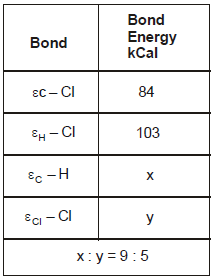From the given data, what is the bond energy of Cl - Cl bond

Detailed Solution for Test: Thermochemistry - Question 12

During bond breakage energy is absorbed and during bond formation it is released. From the reaction we can say that 1 C-H bond is broken 1 Cl-Cl bond is broken 1 c-cl bond is formed and 1 h-cl bond is formed. so using the sign conventions the equation becomes
x+y-84-103= -25 (∆H = -25)
5x=9y ..putting x=9/5y we get y = 57.75 kCal

Test: Thermochemistry - Question 13

From the following data at 25°C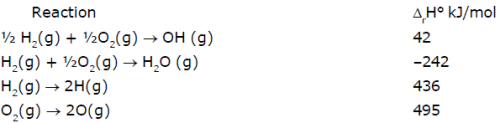Which of the following statement(s) is/are correct :
Statement(a) : ΔrH° for the reaction H2O(g) → 2H(g) + O(g) is 925 kJ/mol
Statement(b) : ΔrH° for the reaction OH(g) → H(g) + O(g) is 502 kJ/mol
Statement(c) : Enthalpy of formation of H(g) is-–218 kJ/mol
Statement(d) : Enthalpy of formation of OH(g) is 42 kJ/mol

Detailed Solution for Test: Thermochemistry - Question 13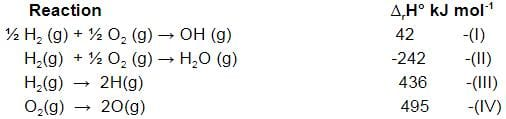Let's check statement (a)
Statement(a) : ΔrH° for the reaction H2O(g) → 2H(g) + O(g) is 925 kJ/mol
For this, we need - (II) + (III) + ½ (IV)
We get, H2O(g) → 2H(g) + O(g) - (-242)+436+½ 495 = 925.5 kJ mol-1
So it is true.
Let's check statement (b)
Statement(b) : ΔrH° for the reaction OH(g) → H(g) + O(g) is 502 kJ/mol
For this we need -(I)+½ (III)+½ (IV)
We get OH(g)   →   H(g)  + O(g)       -(42) + ½ (436) + ½ (495) = 423.5 kJ mol-1
So statement (b) is wrong.
Let's check statement (c)
Statement(c) : Enthalpy of formation of H(g) is -218 kJ/mol
We can see that for enthalpy of formation, we need to divide eqn (III) by 2
So, it would become :-
½ H2(g) → H(g)
436/2 = 218 kJ
So, statement (c) is wrong.
Let's check statement (d)
Statement(d) : Enthalpy of formation of OH(g) is 42 kJ/mol
For that, we have eqn (I) as it is. So, statement (d) is correct.

Test: Thermochemistry - Question 14

The standard molar enthalpies for formation of cyclohexane (l) & benzene (l) at 25°C are -156 & + 49 kJ/mol respectively. The standard enthalpy of hydrogenation of cyclohexane (l) at 25° is -119 kJ mol-1. Use these data to estimate the magnitude of the resonance energy of benzene.

Test: Thermochemistry - Question 15

For hypothetical reaction -

A(g) + B (g) → C (g) + D (g)

Which of the following statements is correct -

Test: Thermochemistry - Question 16

ΔH for CaCO3(s) → CaO(s) + CO2(g) is 176 kJ mol-1 at 1240 K. The ΔU for the change is equal to :

Detailed Solution for Test: Thermochemistry - Question 16

∆H = ∆E + ∆ngRT
176 = ∆E + 1×8.314×1240/1000
∆E = 176 - 10.30 = 165.69 kJ

Test: Thermochemistry - Question 17

Select the correct order in the following :

Detailed Solution for Test: Thermochemistry - Question 17

107 erg = 1 joule and 4.2 joules = 1 cal
Therefore 1 cal > 1 joule > 1 erg

Test: Thermochemistry - Question 18

When enthalpy of reactants is higher than product then reaction will be

Detailed Solution for Test: Thermochemistry - Question 18

The enthalpies of these reactions are less than zero, and are therefore exothermic reactions. A system of reactants that absorbs heat from the surroundings in an endothermic reaction has a positive \(ΔH\), because the enthalpy of the products is higher than the enthalpy of the reactants of the system.

Test: Thermochemistry - Question 19

In the combustion of 4g. of CH4, 2.5 K cal of heat is liberated. The heat of combustion of CHis -

Detailed Solution for Test: Thermochemistry - Question 19

Mol. wt. of methane =16gm
Heat liberated during the combustion of 4gm methane = 2.5kcal
Heat liberated during the combustion of 16gm methane = 2.5/4×16=10kcal
Hence the heat of combustion of methane is 10 kcal.

Test: Thermochemistry - Question 20

Ammonium nitrate can decompose with explosion by the following reaction.

NH4NO3 (s) → N2O (g) + 2H2O ;

ΔH = -37.0 KJ/mol

Calculate the heat produced when 2.50g of NH4NO3 decomposes -

Detailed Solution for Test: Thermochemistry - Question 20

NH4NO3 (s) → N2O (g) + 2H2O ;     ΔH = -37.0 KJ/mol from the above data, we can show that ΔH is for 1 mole of NH4NO3.
For 2.5 g of NH4NO3, we have 2.5/80 = 0.03125 moles
Therefore, heat released = (-37)×(0.03125) = -1.16 kJ

Test: Thermochemistry - Question 21

From the following data, the heat of formation of Ca(OH)2(s) at 18°C is ………..kcal: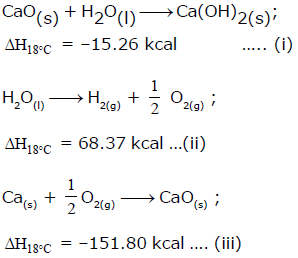Detailed Solution for Test: Thermochemistry - Question 21

The correct answer is Option B.

Ca(s) + O2(g) + H2(g) → Ca(OH)2 , ΔHf = ?

Desired equation = eq (iii) + eq(i) - eq (ii)

ΔHf = (−151.80)+(−15.26)−(−68.37)
ΔHf = (-151.80)+(-15.26)-(-68.37)
ΔHf = −235.43KCalmol−1

Test: Thermochemistry - Question 22

For the process, melting of ice at 260 K the ΔH is -

Test: Thermochemistry - Question 23

Enthalpy of formation of compound is -

Detailed Solution for Test: Thermochemistry - Question 23

This is not fixed. It depends from compound to compound.
A negative enthalpy of formation indicates that the formation of a compound is exothermic---the amount of energy it takes to break bonds is less than the amount of energy that is released when making the bonds.
A positive enthalpy of formation indicates that the formation of a compound is endothermic---the amount of energy it takes to break bonds is greater than the amount of energy that is released when making the bonds.

Test: Thermochemistry - Question 24

The enthalpy of combustion of a substance -

Detailed Solution for Test: Thermochemistry - Question 24

The reason behind is that combustion reactions are always exothermic. So the enthalpy of combustion is always less than zero.

Test: Thermochemistry - Question 25

HA + OH- → H2O + A- + q1 kJ

H+ + OH- → H2O + q2 kJ

The enthalpy of dissociation of HA is

Detailed Solution for Test: Thermochemistry - Question 25

HA + OH⁻ → H₂O + A⁻ + q1 -----(1)
H₂O + q2 → H⁺ + OH⁻ -----(2)
HA + q2 → H⁺ + A⁻  + q1
Hence , bond dissociation energy of HA is (q2 - q1)

Test: Thermochemistry - Question 26

The value of ΔHsol. of BaCl2(s) and BaCl2. 2H2O (s) are – a kJ and b kJ respectively. The value of ΔH Hydration of BaCl2 (s) is-

Detailed Solution for Test: Thermochemistry - Question 26

BaCl2(s) + aq. ⟶ BaCl2 aq.
∆H = -a kJ ------(i)
BaCl2 2H2O(s) + aq. ⟶ BaCl2 aq. + 2H2O
∆H = b kJ -----(ii)
Enthalpy of hydration means that the molecule is just hydeedratt
The reaction should be
(i) - (ii) or
BaCl2(s) + 2H2O ⟶ BaCl2.2H2O       ∆H = -a - b kJ

Test: Thermochemistry - Question 27

A solution of 500 ml of 0.2 M KOH and 500 ml of 0.2 M HCl is mixed and stirred; the rise in temperature is T1. The experiment is repeated using 250 ml of each solution, the temperature raised is T2. Which of the following is true -

Detailed Solution for Test: Thermochemistry - Question 27

Let heat evolved in the 1st case is Q1 and that in the 2nd case is Q2. Then Q2 = 1/2Q1
However, Q1 = 1000T1 and Q2 = 500T2
Therefore, 500T2 = ½ 1000T1 i.e. T1 = T2

Test: Thermochemistry - Question 28

The net heat change in a chemical reaction is same whether it is brought about in two or more different ways in one or several steps. It is known as -

Detailed Solution for Test: Thermochemistry - Question 28

According to Hess law, the thermal effects of a reaction depends upon the initial and final conditions of the reacting substances. It does not depend upon the immediate steps.

Test: Thermochemistry - Question 29

According to Hess's Law the thermal effect of a reaction depends on -

Detailed Solution for Test: Thermochemistry - Question 29

According to Hess law, the thermal effects of a reaction depends upon the initial and final conditions of the reacting substances. It does not depend upon the immediate steps.

Test: Thermochemistry - Question 30

How many kcal of heat is evolved by the complete neutralisation of one mole sulphuric acid with NaOH -

Detailed Solution for Test: Thermochemistry - Question 30

For the reaction of 1 mole of H+ and OH-,we have 13.6 kcal energy released. In H2SO4, we have 2 moles of H+. So for its complete neutralisation, we need 2 moles of NaOH.
So in the end, 2 moles of H+ reacts with2 moles of OH- and 13.6 2 = 27.4 kcal energy is released.

## Chemistry Class 11

204 videos|331 docs|229 tests
 Use Code STAYHOME200 and get INR 200 additional OFF Use Coupon Code